# KR20140132277A - A coefficient error robust transmit feed forward equalization - Google Patents

## Info

Publication number
KR20140132277A
KR20140132277A KR20140052097A KR20140052097A KR20140132277A KR 20140132277 A KR20140132277 A KR 20140132277A KR 20140052097 A KR20140052097 A KR 20140052097A KR 20140052097 A KR20140052097 A KR 20140052097A KR 20140132277 A KR20140132277 A KR 20140132277A
Authority
KR
South Korea
Prior art keywords
equalizer
ffe
data
equation
feed
Prior art date
Application number
KR20140052097A
Other languages
Korean (ko)
Inventor
김병섭
Original Assignee
포항공과대학교 산학협력단
Priority date (The priority date is an assumption and is not a legal conclusion. Google has not performed a legal analysis and makes no representation as to the accuracy of the date listed.)
Filing date
Publication date
Priority to KR20130051427 priority Critical
Priority to KR1020130051427 priority
Application filed by 포항공과대학교 산학협력단 filed Critical 포항공과대학교 산학협력단
Priority claimed from PCT/KR2014/003841 external-priority patent/WO2014182000A1/en
Publication of KR20140132277A publication Critical patent/KR20140132277A/en

• 238000001514 detection method Methods 0.000 claims abstract description 21
• 238000004891 communication Methods 0.000 abstract description 6
• 239000006185 dispersion Substances 0.000 abstract description 2
• 238000010586 diagram Methods 0.000 description 37
• 230000035945 sensitivity Effects 0.000 description 32
• 230000014509 gene expression Effects 0.000 description 31
• 230000000694 effects Effects 0.000 description 16
• 238000001228 spectrum Methods 0.000 description 12
• 230000001702 transmitter Effects 0.000 description 11
• 230000005540 biological transmission Effects 0.000 description 8
• 239000011159 matrix material Substances 0.000 description 5
• 238000000034 method Methods 0.000 description 5
• 239000007787 solid Substances 0.000 description 4
• 238000004458 analytical method Methods 0.000 description 3
• 230000000875 corresponding Effects 0.000 description 3
• 238000005516 engineering process Methods 0.000 description 3
• 206010057190 Respiratory tract infection Diseases 0.000 description 2
• 239000000203 mixture Substances 0.000 description 2
• 229910052710 silicon Inorganic materials 0.000 description 2
• 239000010703 silicon Substances 0.000 description 2
• 238000004088 simulation Methods 0.000 description 2
• 241000255969 Pieris brassicae Species 0.000 description 1
• 210000003491 Skin Anatomy 0.000 description 1
• 230000032683 aging Effects 0.000 description 1
• 230000002238 attenuated Effects 0.000 description 1
• 238000004364 calculation method Methods 0.000 description 1
• 238000004140 cleaning Methods 0.000 description 1
• 230000000295 complement Effects 0.000 description 1
• 230000001276 controlling effect Effects 0.000 description 1
• 230000003247 decreasing Effects 0.000 description 1
• 230000001419 dependent Effects 0.000 description 1
• 229910003460 diamond Inorganic materials 0.000 description 1
• 239000010432 diamond Substances 0.000 description 1
• 238000004519 manufacturing process Methods 0.000 description 1
• 229910044991 metal oxide Inorganic materials 0.000 description 1
• 150000004706 metal oxides Chemical class 0.000 description 1
• 230000000051 modifying Effects 0.000 description 1
• 230000001151 other effect Effects 0.000 description 1
• 239000004065 semiconductor Substances 0.000 description 1
• 238000010206 sensitivity analysis Methods 0.000 description 1
• 238000005549 size reduction Methods 0.000 description 1
• 238000003892 spreading Methods 0.000 description 1

## Images

•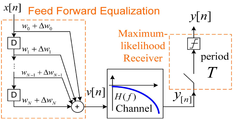•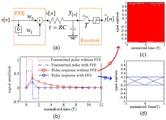•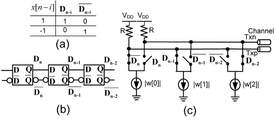••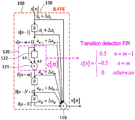••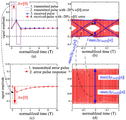•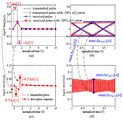•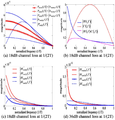••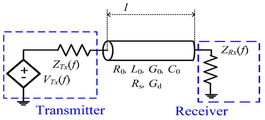•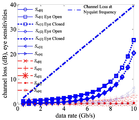••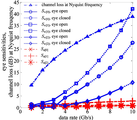•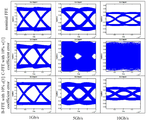## Classifications

• HELECTRICITY
• H04ELECTRIC COMMUNICATION TECHNIQUE
• H04LTRANSMISSION OF DIGITAL INFORMATION, e.g. TELEGRAPHIC COMMUNICATION
• H04L25/00Baseband systems
• H04L25/02Details ; Arrangements for supplying electrical power along data transmission lines
• H04L25/03Shaping networks in transmitter or receiver, e.g. adaptive shaping networks ; Receiver end arrangements for processing baseband signals
• H04L25/03878Line equalisers; line build-out devices
• H04L25/03885Line equalisers; line build-out devices adaptive
• HELECTRICITY
• H04ELECTRIC COMMUNICATION TECHNIQUE
• H04LTRANSMISSION OF DIGITAL INFORMATION, e.g. TELEGRAPHIC COMMUNICATION
• H04L25/00Baseband systems
• H04L25/02Details ; Arrangements for supplying electrical power along data transmission lines
• H04L25/03Shaping networks in transmitter or receiver, e.g. adaptive shaping networks ; Receiver end arrangements for processing baseband signals
• H04L25/03006Arrangements for removing intersymbol interference
• H04L25/03012Arrangements for removing intersymbol interference operating in the time domain
• H04L25/03019Arrangements for removing intersymbol interference operating in the time domain adaptive, i.e. capable of adjustment during data reception
• H04L25/03038Arrangements for removing intersymbol interference operating in the time domain adaptive, i.e. capable of adjustment during data reception with a non-recursive structure
• HELECTRICITY
• H04ELECTRIC COMMUNICATION TECHNIQUE
• H04LTRANSMISSION OF DIGITAL INFORMATION, e.g. TELEGRAPHIC COMMUNICATION
• H04L25/00Baseband systems
• H04L25/02Details ; Arrangements for supplying electrical power along data transmission lines
• H04L25/03Shaping networks in transmitter or receiver, e.g. adaptive shaping networks ; Receiver end arrangements for processing baseband signals
• H04L25/03006Arrangements for removing intersymbol interference
• H04L25/03343Arrangements at the transmitter end

## Abstract

The present invention relates to a coefficient error robust feedforward equalizer, and more particularly to a feedforward equalizer for baseband wireline communication that prevents the influence of a coefficient error caused by dispersion of a nano device.
The coefficient error robust feed forward equalizer according to an embodiment of the present invention includes a receiver 130 that receives input data x according to an integer time index n, A first calculator 110 for summing up the tap signals output from the delay unit D and the N delay units D and a second operator 110 for outputting the data transition value b based on the change of the input data x And a data change detection filter 120.

## Description

{COEFFICIENT ERROR ROBUST TRANSMIT FEED FORWARD EQUALIZATION}
The present invention relates to a coefficient error robust feedforward equalizer, and more particularly to a feedforward equalizer for baseband wireline communications that prevents the effect of a coefficient error.
As the processing capabilities of the digital computing engine have improved and techniques have been developed that use interconnected networks, high bandwidth data transmission is required. The limited channel bandwidth spreads the transmitted pulses at unit intervals, and the received signal is subjected to intersymbol interference.
Feed Forward Equalization (FFE) is a widely used channel compensation scheme for high-speed interconnection in baseband.
CMOS (Complementary Metal-Oxide Semiconductor) integrated circuits with FFE transmitters can achieve high data rates in a limited bandwidth, but due to process distortions, random variables, temperature fluctuations and aging as CMOS technology evolves to nanoscale The variation of the device is increased and a coefficient error is generated largely due to the occurrence of scattering of the nano device, so that the performance of the feedforward equalizer circuit due to the count error is deteriorated and the communication is disturbed.
In addition, there is a problem that the influence of the count error becomes greater in a high-speed operation with a large channel loss.
Therefore, there is a problem in that robustness against counting errors must be ensured in a situation where devices are continuously small and a high data rate is required.
U.S. Published Patent Application 2004/0071219
SUMMARY OF THE INVENTION The present invention has been proposed in order to solve the above problems and provides a feedforward equalizer that operates more like a general structure of a feedforward equalization transmitter and reacts more robustly to a coefficient error There is a purpose.
The coefficient error robust feed forward equalizer according to an embodiment of the present invention includes a receiver 130 that receives input data x according to an integer time index n, A first calculator 110 for summing up the tap signals output from the delay unit D and the N delay units D and a second operator 110 for outputting the data transition value b based on the change of the input data x And a data change detection filter 120.
The data change detection filter 120 is connected between the receiving end and the most delay unit D adjacent to the receiving end and between the two delay units D adjacent to each other among the N delay units D, It is preferable that the first computing unit 110 and the second computing unit 110 are disposed in any one of the delay units D connected in the neighborhood of the first computing unit 110.
The data change detection filter 120 includes a delay unit 122 and a second operator 121 connected to the one delay unit 122. The second operator 121 includes the delay unit 122, (B [nm-1]) based on the previous value (x [n-m + 1]) input to the unit 122 and the current value ]).
The data change detection filter 120 preferably calculates the data transition value based on the following equation.
wherein n and m are integers and n > m is an integer, and n &lt; m &gt;
It is preferable that the data transition value b [n-m] is calculated through logic circuit combination of a plurality of consecutive digital bit values in the data stream.
The data change detection filter 120 is preferably a high pass filter (HPF).
Preferably, the tap signal comprises a feedforward equalizer coefficient (a) and the feedforward equalizer coefficient (a) is adjustable by a user.
The coefficient error robust feed forward equalizer according to the present invention operates in the same manner as a general feedforward equalizer (FFE) in the absence of a count error, and includes a simple logic circuit configuration, it has an effect of improving the robustness.
In addition, the high-pass transition detection filter mounted on the count error robust feed forward equalizer according to the present invention attenuates the perturbation of the signal caused by the count error, the sensitivity of the eye diagram indicating the robustness is improved by about 7 to 17 times.
Also, as the data rate increases and the channel loss increases, the rate of improvement of the eye sensitivity further increases. Therefore, the count error robust feed forward equalizer according to the present invention can be easily applied to a high-speed interconnect.
The effects of the present invention are not limited to those mentioned above, and other effects not mentioned can be clearly understood by those skilled in the art from the following description.
1 is a conceptual diagram of a high-speed interconnect system including a feedforward equalizer in accordance with the prior art.
Figure 2 is an illustration of an example feedforward equalizer in accordance with the prior art;
3 is a conceptual diagram of a conventional 3-tap feed forward equalizer.
4A is a block diagram of a high speed interconnect system including a coefficient error robust feed forward equalizer in accordance with an embodiment of the present invention.
4B is a block diagram specifically illustrating a count error robust feed forward equalizer in accordance with an embodiment of the present invention.
5 is an exemplary diagram of a 3-tap equalizer of a coefficient error robust feed forward equalizer in accordance with the present invention.
FIG. 6 is a graph of a simulation result of a coefficient error effect of a conventional 2-tap feed forward equalizer.
7 is a graph illustrating a simulation result of a coefficient error effect of a 2-tap equalizer of a coefficient error robust feed forward equalizer according to the present invention.
8 is a graph comparing spectra of a feed forward equalizer according to the prior art and a coefficient error robust feed forward equalizer according to the present invention.
9 is a graph illustrating the eye sensitivity of a conventional two-tap feed forward equalizer for a primary RC channel and a coefficient error robust feed forward equalizer in accordance with the present invention.
10 is a diagram illustrating an embodiment of a lossy transmission line channel model.
11 is a graph showing the eye sensitivity and channel loss at the Nyquist frequency of a 5-tap feed forward equalizer in accordance with the prior art and a feed forward equalizer in a 40 cm PCB channel.
FIG. 12 is a graph showing an eye diagram for each data transmission rate in FIG.
13 is a graph showing the eye sensitivity of a prior art 3-tap feed forward equalizer in a 3.5 cm silicon interposer package and a feed forward equalizer in accordance with the present invention.
14 is a graph showing an eye diagram for each data transmission rate in FIG.
Hereinafter, a count error robust feed forward equalizer according to the present invention will be described in detail with reference to the drawings. In the drawings, the same reference numerals are used to designate the same or similar components throughout the drawings. In the following description of the present invention, a detailed description of known functions and configurations incorporated herein will be omitted when it may make the subject matter of the present invention rather unclear.
A conventional feedforward equalizer according to the prior art for a high speed interconnect comprises a channel, a feedforward equalization transmitter (FFE Tx) and a 1-bit quantization receiver (Rx) as in FIG. 1, Cable, coaxial cable, backplane, PCB, package, and on-chip wires, with channels ranging from a few centimeters to tens of meters.
When the data rate is in the range of several Gb / s to several tens of Gb / s, the channel has the characteristics of a low-pass filter (LPF) and inter-symbol interference (ISI) .
If the channel loss is large at the Nyquist frequency, inter-signal interference seriously disturbs the communication. Generally, it is known that the loss is small when the channel loss is 0 to 10 dB, is large when the channel loss is 10 to 20 dB, and is very large when the channel loss is 20 to 30 dB. Therefore, channel loss exceeding 30dB is very rare and is known to be extremely difficult to communicate. There is a need to design a feedforward equalization (FFE) transmitter that can ensure reliability under these circumstances.
Referring to FIG. 1, a feedforward equalization transmitter (FFE Tx) has been used as a means to compensate for channel loss to ensure data rate.
The feedforward equalizer (FFE) generally has a tap coefficient w = [w 0 w 1 ... w N ] T and coefficient error Δ w = [Δw 0 ? W 1 ... Is represented by a finite impulse response filter having [Delta] w [ N ] T.
Generally, two to five taps are suitable, and a feed forward equalizer (FFE) having an appropriate tap coefficient w value multiplies the signal y [n] arriving at the receiver Rx by a 2-level (HPF) that compensates for channel loss to produce a pulse amplitude modulation (PAM2) signal. y [n] corresponds to the value arrived at the receiver x [nm] where x [n] is the transmitted data sequence and the signal level is 1 (bit '1') or -1 (bit '0') I have. In this case, m denotes a delay time until x [n] reaches the receiver through the channel.
Typical receivers typically rely on eye diagrams obtained through a feedforward equalizer (FFE) because complex coding techniques in these interconnects are expensive and do not have large white noise magnitudes.
Therefore, minimizing the inter-signal interference (ISI) and maximizing the eye diagram are important factors for improving the efficiency of the interconnect system.
Figure 2 (a) is an illustration of the simplest and most common 2-tap FFE structure.
The wire is modeled as a primary RC circuit with a time constant τ.
The continuous-time pulse response h (t) of the system is given by h (t), u (t) (1-e -t / τ ), where u (t) is the unit step function. Referring to FIG. 2 (b), the continuous time pulse response of this system is shown in a circle and shown as a solid line (Pulse response without FFE).
The discrete-time pulse response h [n] of this channel is equal to h (nT) and can be expressed by the following [Equation 1].
[Equation 1]
h [n] = 0 if n≤0 , or h [n] = (1-e -T / τ) e - (n-1) T / τ if n> 0
n is an integer time index.
h [n]: discrete time impulse response
T: data period
τ: time constant
2 (b), the impulse response h (t) of the system in which the feedforward equalizer (FFE) is not mounted is smaller than the time constant (tau) in the feedforward equalizer (FFE) (ISI tail), which is longer than the impulse response of the system with the ISI.
According to Equation 1, h [n] decreases exponentially with h [n-1] e -T /? In the case of n? 1, The inter-signal interference (ISI) can be completely eliminated through a 2-tap FFE with a feedforward equalizer coefficient (optimal FFE coefficient).
[Equation 2]
w opt = [1 / (1 + e -T / τ) -e -T / τ / (1 + e -T / τ)] T
w opt : optimized FFE coefficient
T: data period
τ: time constant
When the unit impulse function [delta] [n] is input as x [n], the feedforward equalizer FFE multiplies the transmitted pulse to remove the ISI tail, Undershoot like a long dotted line. As a result, the discrete-time pulse response g [n] of the system equipped with the feedforward equalizer (FFE) is not affected by inter-signal interference as shown by the diamond shape and the solid line in Fig. 2 (b) the eye diagram is completely opened as shown in (d).
The optimal value g opt [n] of the discrete time pulse response g [n], defined as h [n] * w [n], by the characteristics of the Linear Time Invariant (LTI) .
[Equation 3]
g opt [n] = 1 - e - T / τ / 1 + e - T / τ if n = 1, or g [n] = 0 if n ≠ 1.
If the feedforward equalizer (FFE) is not mounted, referring to FIG. 2 (c), the eye diagram is closed, indicating that the feedforward equalizer (FFE) can improve the eye diagram have.
Least-square-error (LSE) is used to determine the feedforward equalizer (FFE) coefficients in various channels. The feedforward equalizer (C-FFE) coefficient w lse [n] according to the prior art which is a solution of the least squares method minimizes the distance between g [n] (= h [n] * w [n]) and δ [nm] And is represented by the following [Expression 4].
[Equation 4]
Using the vector and the matrix representation to obtain the solution of the least squares method, the solution of [Equation 4] can be approximated as [Equation 5]. w lse and h are vectors and are truncated vector representations of w lse [n] and h [n], respectively. h k and δ -m are column vectors with n [n + kl] and δ [nm-1] as the nth element, respectively. And h is H = [ h h -1 h -2 ... h -n ].
[Equation 5]
Generally, the maximum value | v [n] | of the signal transmitted from the feedforward equalizer (FFE) transmitter shown in FIG. 1 has the same constraint as the following Equation (6). Therefore, w lse [n] is normalized as [Equation 7] to satisfy Equation .
[Equation 6]
max | v [n] | = | w  | + | w  | + | w  | + ... + | w [N] |? 1
Therefore, to satisfy [Equation 6], w lse [n] can be normalized as in Equation 7 below.
[Equation 7]
In describing an embodiment of the present invention, a conventional feedforward equalization transmitter (conventional FFE) is referred to as C-FFE for convenience, and an equalization transmitter (robust-FFE) that prevents the count error effect of the present invention is referred to as B-FFE .
The hardware implementation of the prior art feedforward equalizer (C-FFE) and the coefficient error associated therewith is as follows.
3 shows a prior art three-tap feed forward equalizer transmitter structure, wherein the flip-flop or latch shown in FIG. 3B is a delay unit (delay unit) of FIG. 1, D). The output of the latch Represents x [ni], and its value is determined according to the truth table of Fig. 3 (a).
The differential current mode logic circuit (CML) of FIG. 3 (b) And the output values of the latches are added to the output nodes Txp and Txn to calculate the sum of w [i] x [ni] as shown in the following equation . Here, V Tx between Txp and Txn is thevenin-equivalent differential voltage corresponding to v [n] in FIG.
[Equation 8]
V Tx = (V dd -RI Txp ) - (V dd -RI Txn) = R (I Txn -I Txp)
= R (w  x [n] + w  x [n-1] + w [
At this time, if V Tx is too large, there is a problem that the transistor operates in an undesired state. Therefore, the same restriction condition as in the above-mentioned [Equation 6] is required.
The transmitter of the feedforward equalizer is vulnerable to a coefficient error caused mainly by variations of nano elements.
That is, the current source and the coefficient | w [n] | shown in FIG. 3 (c) are sensitive to the scatter generated by the nanoscale technology, and such scattering is not easy to control in techniques below micrometers There is a problem that robustness can not be secured.
As shown in Fig. 1, the influence of scattering of the nano device can be modeled as a constant random variable Δ w added to the coefficient w . These phenomena occur much more slowly than data communication over a period of several minutes to several years due to the manufacturing process, changes in circuit operation, and the like, which occur during transmission The random error modeling such as Δw is suitable for the counting error.
Hereinafter, the feedforward equalizer 100 according to an embodiment of the present invention will be described in detail with reference to FIGS. 4A and 4B. 4A is a block diagram of a high-speed interconnect system including a coefficient error robust feed forward equalizer 100 (B-FFE) in accordance with an embodiment of the present invention, and FIG. 4B is a block diagram of a coefficient error FIG. 2 is a block diagram specifically showing a robust feed forward equalizer 100 (B-FFE).
4A, a high-speed interconnect system including a coefficient error robust feed forward equalizer 100 (B-FFE) according to an embodiment of the present invention includes a feedforward equalizer 100 (B-FFE) A receiver 300 for receiving the output of the feedforward equalizer 100 (B-FFE) and a channel 200 for communication between the feedforward equalizer 100 (B-FFE) and the receiver 300 .
In the case of the receiver 300 and the channel 200, the coefficient error robust feed forward equalizer 100 according to the embodiment of the present invention will be described with reference to FIG. 4B, B-FFE) will be described in detail.
The coefficient error robust feed forward equalizer 100 (B-FFE) according to an embodiment of the present invention includes a receiving end 130, N delay units D, a first calculator 110, And a data change detection filter 120. The receiver 130 receives the input data x according to the integer time index n, where x [n] is the transmitted data sequence with a signal level of 1 (bit '1') or -1 (bit ' 0 '). In this case, m denotes a delay time until x [n] reaches the receiver through the channel. The first calculator 110 is connected in series to the receiving end 130 of the N delay units D and performs a function of summing the tap signals output from the N delay units D, respectively. Lastly, the data change detection filter 120 performs a function of outputting the data transition value b based on the change of the input data x. Hereinafter, the data change detection filter 120 will be described. A coefficient error robust feed forward equalizer 100 (B-FFE) according to an embodiment will be described.
The data change detection filter 120 in the coefficient error robust feed forward equalizer 100 (B-FFE) according to an embodiment of the present invention includes the N delay units D as shown in FIG. 4B, The first delay unit D or the first calculation unit 110 connected to the receiving end 130 and the receiving end 130 in the neighborhood of the delay unit D and the first delay unit D, 1 operator 110 and the rearmost delay unit D connected adjacent thereto.
Specifically, the data change detection filter 120 is composed of one delay unit 122 and a second operator 121 connected to the one delay unit 122. Particularly, Based on the previous value x [n-m + 1] input to the delay unit 122 of the delay unit 122 and the current value x [nm] output from the one delay unit 122 [nm]).
The process of calculating the data transition value by the data change detection filter 120 will be described in more detail. In FIG. 4B, x [n] is input data, and b [nm] is a value representing a data transition of x [n]. C [n] is a high-pass filter (HPF) that detects the change of data, c [m-1] is 0.5, c [m] is -0.5, and c is any integer that is not m- n] is zero. That is, c [n] is defined as a value that converts x [n-m + 1] to b [nm]
[Equation 9]
b [n-m] = 0.5x [n-m + 1] -0.5x [n-m] = c [n] * x [n].
x [n]: input data
b [n-m]: Transition of input data
c [n]: Data change detection high-pass filter
b [nm] may have a value of -1, 0, 1, and when b [nm] is -1, x [n] means a change from 1 to -1, and b [nm] , It means that x [n] changes from -1 to 1, and when b [nm] is 0, it means that there is no change of x [n]. That is, b [n-m] includes information that x [n] changes.
As a result, the data transition value computed and output according to Equation (9) becomes -1 when the data input to the data change detection filter 120 is changed from 1 to -1, and when the input data changes from -1 to 1 1, and becomes 0 if there is no change in the input data.
In addition, the coefficient error robust feed forward equalizer according to an embodiment of the present invention is capable of calculating the data transition value b [nm] through logic circuit combination of a plurality of consecutive digital bit values in the data stream .
Figure 5 (a) is a truth table representing the expression of [Formula 9] of the 3-tap coefficient error robust feed forward equalizer (FFE-B) according to an embodiment of the invention, two digital bit (D pn - i-1 , D nn -i-1 ). At this time, the two digital bits D pn-i-1 and D nn -i- 1 is configured as shown in Figure 5 (b) using the AND gate.
FIG. 5C is a circuit diagram of a circuit for calculating a product of addition and coefficient of a 3-tap coefficient error robust feed forward equalizer (B-FFE) according to an embodiment of the present invention. Equalizer (C-FFE) It is a circuit of the same type as a CML circuit. Since the cost of the AND gate in the nanoscale CMOS technology is very low, there is an economical effect in constructing the B-FFE according to the present invention.
In the coefficient error robust feed forward equalizer 100 (B-FFE) according to the embodiment of the present invention, tap signals are output from N delay units D as described above, The feedforward equalizer coefficient (a) is preferably configured to be adjustable by a user, which may include a steady state condition (a) and a steady state error coefficient (C-FFE) according to the prior art and the coefficient error robust feed forward equalizer (B-FFE) according to an embodiment of the present invention can be mathematically matched to each other .
In order to explain this in detail, when cleaning up the [formula 9] the above-described [Equation 9] using the vector and the matrix representation can be represented with b [n] as B x x [n], wherein b [n] = [x [n] x [n-1] ... x [n-m + 1], b [nm] b [nm-1] b [nN]] T , x [n] = [x [n] x [n-1] x [n-2] x [nN]] T , and B x is defined as in Equation 10 below. (B x is N + 1 by N + 1 matrix, B x The identity matrix at the top left is a matrix of m by m.)
[Equation 10]
On the other hand, v of the feed forward equalizer (C-FFE) according to the above-described prior art [n] is because x [n] T w, v [n] = b [n] T a = x [n] T B x T a, and v [n] = x [n ] T using the w, no feed-forward equalizer (C-FFE) any coefficient error robust feed-forward in accordance with the invention equalizer (B-FFE) according to the prior art; And a = [a 0 a 1 ... a n ] T can be derived as shown in [Expression 11] and [Expression 12] below.
[Equation 11]
w = B x T a
[Equation 12]
a = (B x T ) -1 w
The feedforward equalizer coefficient a of the feedforward equalizer 100 (B-FFE) according to an embodiment of the present invention can be calculated using Equation 11 and Equation 12 using a conventional feedforward equalizer FFE) according to an embodiment of the present invention can be mapped to the feedforward equalizer coefficient w of the conventional feed forward equalizer (C-FFE) ) Contrast Coefficient It is possible to obtain not only the effect of being strong against error but also the function of all conventional feedforward equalizer (C-FFE) can be implemented as it is.
As described above, the scattering effect of the element generated in the coefficient error robust feed forward equalizer (B-FFE) according to the present invention is such that the constant random coefficient error? A is smaller than the feed forward equalizer coefficient a Lt; / RTI &gt;
Hereinafter, robustness of a coefficient error robust feed forward equalizer (B-FFE) according to the present invention will be described. A 2-tap coefficient error robust feed forward equalizer in a first order RC channel according to an embodiment of the present invention will be described as an example.
Since the feedforward equalizer (FFE) is an LTI system, it can analyze the effect of a count error from the perburbation of the pulse response.
By substituting 0 for the nominal coefficient of the feedforward equalizer (FFE), the perturbation y [n], y [n], can be obtained. That is, by replacing w [n] shown in FIG. 1 with 0, it is possible to derive [Expression 13] for the feed forward equalizer (C-FFE) according to the prior art.
[Equation 13]
H ? W [m] [n], which is defined as ? W [m] h [nm] in Equation 13 above is the influence of the coefficient error Δw [m] on Δy [n] .
Here, the reference to [formula 13], Δy [n] is the coefficient error because the values adding up all the influence of Δw [m], the perturbation of the feed forward equalizer (FFE) system all h as a normal pulse response Δw [m] [n]. Therefore, h ? W [m] [n] can be defined as a coefficient error pulse by? W [m]. Referring to FIG. 4, the pulse response change Δy [n] of the coefficient error robust feed forward equalizer (B-FFE) according to the present invention can be derived as:
[Equation 14]
In Equation (14), h ? A [m] [n] is a coefficient error pulse response by? A [m]. When 0≤k≤m-1 h Δa [k] [n] is a Δa [k] h [nk], when m≤k≤N h Δa [k] [n ] = Δa [k] h [ nk] * c [n] = 0.5? a [k] (h [nk-m + 1] -h [nkm]).
6 is a diagram showing the result of simulating the influence of? W  on a 2-tap C-FFE according to the prior art mounted to compensate for loss of the primary RC channel. 6 (a), the pulse response without inter-signal interference (ISI) can be confirmed. As a result, as shown by the solid line in FIG. 6 (b) eye diagram.
However, if the pulse to which the count error? W  is transmitted and the response is slightly changed from the steady state as in 2 or 4 of Fig. 6 (a), the spread of the upper and lower portions of the eye diagram A phenomenon occurs. That is, the maximum value of the eye diagram spread generated in FIG. 6 (b) is expressed by Δy Δw  [n] expressed by Δy [n] Is equal to the maximum value of the eye diagram of FIG.
If you define the height of the child (eye) decreases when the only error of Δw  to Δv eye, Δw , Δv eye, Δw  is 2max | represented by | Δy Δw  [n ] .
As shown in Figure 6 (c), h Δw [ 0] [n] is because RC response exponentially decreasing, max | Δy Δw  [n] | is for all the n x [n] = 1 or -1. Assuming that the error rate is? 0 , the coefficient error? W  can be represented by? 0 w , so that the following equation can be obtained from [Expression 1], [Expression 2], [Expression 13] max |? y ? w  [n] |.
[Equation 15]
max [Delta] w  [n] | = max |
= | Δw  | Σ∀ n h [n] = | Δw  | = | α 0 | / (1 + e -T / τ)
Further, when the definition of? W  is applied to? W , max |? Y ? W  [n] | can be derived as shown in the following equation.
[Equation 16]
max | Δy Δw  [ n] | = max | Δw  h [n-1] * x [n] | = | Δw  | Σ∀ n h [n]
= | Δw  | = | α 1 | eT / τ / (1 + e- T / τ )
In Fig. 6 (d),? W  is -20% of w  which is 0.6 at -0.12. Thus, when T is 0.4?, Max |? Y? W  [n] | becomes 0.12 and the height of the reduced eye? V eye ,? W  = 0.4-0.16 = 2max |? Y? W  [n] | = 0.12? 2 = 0.24.
Fig. 7 is a graph showing a case where a coefficient error robust feed forward equalizer (B-FFE) according to the present invention is mounted instead of the feedforward equalizer (C-FFE) according to the prior art in Fig. 6, a ) in the first embodiment. In the steady state, the operation of the feedforward equalizer (C-FFE) according to the prior art and the coefficient error robust feed forward equalizer (B-FFE) according to the present invention are the same, It is possible to obtain a pulse response without ISI and referring to the solid line in Fig. 7 (b), it can be seen that there is no spreading of the eye diagram.
Referring to FIG. 7 (a), Δa  changes the pulse response and causes the eye diagram to spread as shown by the dotted line in FIG. 7 (b).
However, since the coded error robust feed forward equalizer (B-FFE) according to the present invention operates as a high-pass filter (HPF) shown in FIG. 4, c [n] , The perturbation of the transmitted pulse is modified to reduce h [Delta] a [ 1] [n].
As a result, comparing Fig. 7C and Fig. 6C, h ? A  [n] in Fig. 7C is significantly lower than h ? W  [n] It can be seen that the spread of the eye diagram in Figs. 7 (d) and 7 (b) is much smaller than that in Figs. 6 (d) and 6 (b).
Wherein when the [formula 15] and the error rate in analogy to α n in the Formula 16] assumed to be β n using that Δa [m] = β n a [m], as follows: [Formula 17] and [formula 18] h [Delta] a [m] [n].
[Equation 17]
[Equation 18]
c [n] does not change h ? a  [n]. Therefore, h Δa  [n ] is h Δw  [n] , h Δw  [n] the same decreases exponentially with, and reduce the perturbation caused in the h Δa  [n] The effect does not appear. However, since the steady state value a  has a small value as shown in [Equation 19], | Δa  | is larger than | Δw  |, | Δw  |, and | Δa [ Generally, it has a much smaller value.
[Expression 19]
H (f): Frequency response of the channel
f N : Nyquist frequency
[Equation 19] can be derived as follows. First, x [n] = '... , 1, -1, -1, -1, 1, ... 'Makes a square wave of v (t) (a continuous-time signal corresponding to v [n]). Therefore, v 1st (t) = 4 sin (2fNt) / π, which is the first harmonic signal (1 st harmonic) of v (t) Since the channel is a low-pass filter (LPF), at the receiver, y 1 st (t) (the first harmonic signal of the continuous-time signal y (t) corresponding to y [n]) dominates. When the channel distortion is well compensated, | y 1st (t) | ≒ a so approximation is possible to  is the y 1st (t) ≒ a [ 0] (2πf N t) sin. At this time, at the Nyquist frequency f N , | y 1 (t) | = | v 1 (t) || H (f N ) |
According to the above equation , a  value can be predicted within an error of about 20% and a  is much smaller than other coefficients. In this embodiment, | Δw  | is 0.12, | Δw  | is 0.08, | Δa  | is larger than that for the same 20% Is 0.16.
The worst perturbation as shown in the following [Expression 20] and [Expression 21] is performed by using Expression 14, Expression 17 and Expression 18 expressing the influence of DELTA a  and DELTA a [ the worst perturbation value max |? y ? a  [n] | And max |? Y? A  [n] |.
[Equation 20]
0 [n] | = max |? a  h [n] * x [n] |
= | Δa  | Σ∀ n h [n] = | Δa  | = | β 0 | (1-e -T / τ) / (1 + e -T / τ)
[Equation 21]
max | Δy Δa  [ n] | = max | Δa  h [n] * c [n] * x [n] |
= 0.5 | Δa  | {h  -Σ∀ n≥2 (h [n] - h [n-1])} = | Δa  | (1-e -T / τ)
= 2 | β 1 | e -T / τ (1-e -T / τ) / (1 + e -T / τ)
7, the Δa  is 0.16, the max | Δy Δa  [n] | is approximated to 0.05, and the eye size is approximately 0.29. This value is 80% higher than the value of 0.16 in FIG. Further, max |? Y? A  [n] | is calculated as max |? Y ? W  [n] (= 0.12) 56%, max |? Y ? W  [n] | (= 0.08).
A method for improving the robustness of a coefficient error robust feed forward equalizer (B-FFE) according to the present invention, which is explained through the analysis of the frequency domain, is as follows.
Referring to FIG. 1, the continuous-time perturbation function p ? W [m] (t) of a pulse transmitted by ? W [m] is derived as in Equation 22 below, The spectrum (P ? W [m] (f)) of p ? W [m] (t) when ? [N] is input as x [n]
[Equation 22]
p ? w [m] (t) =? w [m]? T (t-mT)
[Equation 23]
P Δw [m] (f) = Δw [m] Λ T (f) e - j2 π fmT = Λ Δw [m], T (f) e - j2 π fmT
In Equation 22, Π T (t) is defined as Π T (t) = 1 when 0 ≤ t <T and Π T (t) = 0 for all other t.
In the [formula 23], Λ T (f) = Tsinc (fT) e -jπ fT is the Fourier transform of Π T (t) Λ Δw [ m], T (f) = Δw [m] Λ T (f )to be. Further, the continuous time channel response h ? W [m] (t) and its spectrum H ? W [m] (t) of p ? W [m] (t) are obtained from h (t), the above- f) is derived as shown in the following equations (24) and (25).
[Equation 24]
h ? w [m] (t) = (h * p ? w [m] ) (t)
[Equation 25]
H Δw [m] (f) = H (f) P Δw [m] (f) = H (f) Λ Δw [m], T (f) e -j2πfmT
The [formula 25] in Λ Δw [m], T ( f) is with respect to a sequence of x [n] = δ [n] Δw [m] and x (t) = Π T ( t) Π Δw caused by [m] and T (t) = Δw [m] Π T (t). Therefore, Π Δw [m], T (t), Λ Δw [m], and T (f) are defined as input error pulses and their spectra by Δw [m].
On the other hand, the continuous-time transmission error pulse p ? A [m] (t) by? A [m] in the coefficient error robust feed forward equalizer (B-FFE) according to the present invention and its spectrum are given by [ Is derived as shown in [Equation 27].
[Equation 26]
[Equation 27]
In this case, c (t) = 0.5δ ( t) -0.5δ (tT) is the continuous-time impulse response of c [n], C (f ) = jsin (πfT) e (-jπ fT) is c (t ) &Lt; / RTI &gt; Since the continuous time count error pulse response hΔa [m] (t) by Δa [m] is (h * p Δa [m] ) (t), H (f) and P Δa [m] the spectrum H ? a [m] (f) of h ? a [m] (t) can be derived as shown below.
[Equation 28]
The common expressions Λ Δcoeff [m], T (f) = Δ coeff [m] Λ T (f) through the above expressions 23, 25, 27, Is the spectrum of the input error response of the input reference by Δ coeff [m] when Π T (t) is input as the input pulse. Therefore, Λ Δcoeff [m] and T (f) are both sinc functions in the feed forward equalizer (C-FFE) according to the prior art and the coefficient error robust feed forward equalizer (B-FFE) according to the present invention. Λ Δw [m], T (f) for the feed-forward equalizer (C-FFE) of the prior art is compared to that filtered only by H (f) as shown in the [formula 25], the coefficient according to the invention In the error robust feed forward equalizer (B-FFE), ΛΔa [n] and T (f) (n ≠ 0) are converted into a high pass filter (HPF) C (f) ) Channel H (f). Therefore, the coefficient error robust feed-forward equalizer (B-FFE) according to the invention H Δa [n] (f) (n ≠ 0) is a feed forward equalizer (C-FFE) in accordance with the prior art H Δw is much weaker than [n] (f), and Λ Δa  and T (f) when n is 0 have values much smaller than other values, as shown in [Expression 19].
8 (a) to 8 (c) show a two-tap feed forward equalizer (C-FFE) according to the prior art and a coefficient error robust according to the present invention in a primary RC channel in which a loss of 18 dB occurs in f N A graph comparing various spectra of a feedforward equalizer (B-FFE).
Referring to Figure 8 (a), the coefficient error pulse spectrum LAMBDA of the input stage reference of the prior art feed forward equalizer (C-FFE) and the count error robust feed forward equalizer (B-FFE) Δw , T (f ), Λ Δw , T (f), Λ Δa , T (f), and Λ Δa , T ( f)) is the lowest frequency energy It has a concentrated sinc function form. 8 (b) shows a spectrum of C (f) which is a high-pass filter (HPF). C (f) is less than P Δw  (f) and P Δw  (f) As shown in 8 (a) also attenuated the amount of the low frequency P Δa  (f) Size. Referring to Fig. 8 (b), the spectrum of H (f) sharply decreases as the frequency increases. Thus, as shown in the [formula 28] C (f) H ( f) overall has a smaller size in the frequency range, As shown in FIG. 8 H Δa  (f ) is H Δw  (f) or H is much smaller than Δw  (f). The H ? A  (f) not filtered by the high pass filter (HPF) C (f) is also smaller than H ? W  (f) or H ? W  (f) .
The coefficient error robust feed forward equalizer according to the present invention is effective when channel robustness is important due to a large dispersion of channels. Fig. 8 (d) shows the result of simulating the spectrum of Fig. 8 (c) in a 36 dB channel with a larger loss. H Δa  (f) and H Δa  (f) is 8 than in the 8 (c) also when compared with H Δw  (f) and H Δw  (f) ( d), it can be seen that the value is smaller. Therefore, the coefficient error robust feed forward equalizer (B-FFE) according to the present invention provides a much better improvement effect in a lossy channel.
Eye diagrams are widely used to measure the quality of communication. Thus, in order to quantify the robustness of the feedforward equalizer (FFE), the eye sensitivity to an n-th feed forward equalizer (FFE) coefficient κ [n] k [n] . S k [n] is obtained by dividing the reduction rate of the eye height reduction value Δv eye, Δκ [n] by Δκ [n] from the optimal eye height v eye by the error rate of κ [n] , Where the other coefficient error is replaced by zero.
[Equation 29]
If the eye sensitivity of the feedforward equalizer (FFE) is large, the eye diagram of the feedforward equalizer (FFE) is more sensitive to counting errors. Thus, eye sensitivity is useful as a measure of robustness.
A 2-tap feedforward equalizer (FFE) for the primary RC channel has a theoretically perfect eye in the absence of any perturbation and has a child height (g) according to FIG. 6 and g opt [n] v eye ) is determined by 2g opt  as in the following [Expression 30].
[Equation 30]
v eye = 2 (1 - e - T / τ ) / (1 + e - T /
? W [m] = -2max |? Y? W [m] [n], as described above. Thus, w  and w  has the same sensitivity as the following [Expression 31] and [Expression 32].
[Equation 31]
[Equation 32]
T << πτ a case, the [equation 31] and [equation 32] is a Taylor series (e -T / τ ≒ 1- T / τ) and a channel frequency response at f N (H (f N) = 1 / (1 + j2? F N ?)? T / (j??) By the following equations (33) and (34).
[Equation 33]
[Equation 34]
9 shows the eye sensitivity of the prior art 2-tap feed forward equalizer (C-FFE) for a primary RC channel and the count error robust feed forward equalizer (B-FFE) f N ). Referring to FIG. 9, it can be seen that the above equations (33) and (34) are similar to the equations (31) and (32), respectively.
As the denominator (1-e -T / τ ) converges to zero, the channel loss and S w  and S w  towards almost infinite linearly . For T = τ, the channel loss is 10 dB, S w  = 1.58, and S w  = 0.58. On the other hand, when T = 0.1τ, the channel loss is 30dB, S w  = 10.5, and S w  = 9.5. That is, when T = 0.1τ, the worst eye sensitivity is increased by 6.6 times as compared with T = τ.
In general, if the data rate is fast and the channel loss is large, the eye sensitivity is also very large, and the high eye sensitivity means that the count error amplifies. Thus, even a very small count error can cause the eye diagram to be closed, so designers must strictly control variations in coefficients even when they consume excessive hardware area and power.
A w  error of only 1.9% can reduce the height of the eye by 20%, and if T = 0.1, 12.6% w  Reduce. Therefore, the design cost of the FFE is high in a very lossy channel with a channel loss of 30 dB or more.
The count error robust feed forward equalizer (B-FFE) according to the present invention has the effect of improving eye sensitivity in a channel with a very high loss. The optimal error robust feed forward equalizer (B-FFE) coefficient a opt for the primary RC channel according to the present invention is calculated using Equation (2) and Equation (12) .
[Equation 35]
a opt = (B x T) -1 w opt = [(1-e -T / τ) / (1 + e -T / τ) 2e -T / τ / (1 + e -T / τ)] T
The eye sensitivity of the coefficient error robust feed forward equalizer (B-FFE) according to the present invention is derived from h ? A [m] [n] of [Expression 17] and [Expression 18]. Is | Δy Δa  [n] | | the [formula 14] and [formula 17] are from max | can be seen that, Δv eye = -2max | | Δa  Δy Δa  [n] Therefore,? V eye = -2 |? A  |. Also, v is an eye = 2g opt [n] and g opt [n] = a [ 0] , because it is eye v 2a = . Therefore, the eye sensitivity of [ Delta] a  (S a  ) can be derived by [Equation 36].
[Equation 36]
Figure 9 shows that S w  and S w  are increased but S a  = 1 when the data rate increases. Therefore, even if the data rate is very high, the influence of [Delta] a  is not amplified by the eye sensitivity of the count error robust feed forward equalizer (B-FFE) according to the present invention.
max | Δy Δa  [ n] | = | Δa  | (1-e -T / τ) is derived from the above [formula 14] and [formula 18], Δv eye, Δa [ 1] = 2max | Δy Δa  [n] | = 2 | Δa  | (1-e -T / τ ). If the Taylor series approximation is applied to [Expression 30] and [Expression 35], S a  is simplified as shown in Expression (37) below.
[Equation 37]
As can be seen from FIGS. 9 and 37, when the data rate increases, S a  increases but converges to '2'.
9 is a graph showing the eye sensitivity of a prior art feedforward equalizer (C-FFE) according to the data rate and a count error robust feed forward equalizer (B-FFE) according to the present invention. The sensitivity of the feed forward equalizer (C-FFE) according to the prior art increases toward infinity and the sensitivity of the count error robust feed forward equalizer (B-FFE) according to the present invention converges to 1 or 2 Able to know.
Thus, the difference in eye sensitivity, which is the degree of improvement in the robustness of the prior art feed forward equalizer (C-FFE) and the inventive count error robust feed forward equalizer (B-FFE), increases infinitely . (B-FFE) according to the present invention when the channel loss is 10 dB (τ / T = 1), 30 dB (τ / T = 10), and 40 dB The worst eye sensitivities are S a  = 1, S a  = 1.8, and S a  = 1.9. This result is 1.54 times, 5.9 times, and 17 times the worst case sensitivity of the feedforward equalizer (C-FFE) according to the above-mentioned prior art, 1.54, 10.6, and 32.3, respectively .
Hereinafter, in order to clarify the eye sensitivity analysis, the eye sensitivity of a feedforward equalizer (C-FFE) according to the prior art and a coefficient error robust feed forward equalizer (B-FFE) Numerical analysis will be explained.
The actually used channel can be modeled as a lossy transmission line as shown in FIG. The transfer function of the lossy transmission line is expressed by the formulas 38, 39, and 40 using the telegrapher's equation including the telegrapher equation and the frequency-dependent secondary impact of the channel. , And [Expression 41].
[Expression 38]
[Equation 39]
[Equation 40]
[Equation 41]
(F) is the frequency response of the channel of length l, Z c (f) is the characteristic impedance of the channel, and Z Tx (f) is the channel impedance of the channel. In the above Equation 38, 39, 40 and 41, (f) and Z Rx (f) are the termination impedances of the transmitter and the receiver, respectively, R 0 , L 0 , G 0 , and C 0 are the RLGC parameters of the channel at DC, R s and G d are the skin effect, Respectively. The frequency response of the PCB or the package wire can be mathematically calculated from the above-mentioned [Expression 38], [Expression 39], [Expression 40] and [Expression 41].
11 shows the eye sensitivity of a 5-tap feed forward equalizer (C-FFE) according to the prior art and a 5-tap coefficient error robust feed forward equalizer (B-FFE) according to the present invention in a 40- And the channel loss at the Nyquist frequency.
To calculate the eye sensitivity, we first mathematically calculate the eye height reduced by the 10% count error. The worst case analysis is used for sequence lengths with a bit-error-rate (BER) of 10 -18 . Several closed eyes are indicated by the dotted lines in Fig. In order to describe a closed eye diagram, the difference between the minimum signal size of bit '1' and the maximum signal size of bit '0' is defined as the height of the child. If the child is closed, the height of the defined child is negative, Indicates how much worse the signal level should be improved to open the eye diagram.
Referring to FIG. 11, the eye sensitivity of the count error robust feed forward equalizer (B-FFE) according to the present invention is much smaller than that of the prior art feed forward equalizer (C-FFE). The worst case sensitivity (S a  ) of the coefficient error robust feed forward equalizer (B-FFE) according to the present invention is 3.55 when the Nyquist channel loss is about 40 dB and the data rate is 10 Gb / , And the eye sensitivity (Sw ) of the feedforward equalizer (C-FFE) according to the prior art is 25.7. That is, according to the present invention, there is an effect of improving the eye sensitivity by about 7 times or more as compared with the feed forward equalizer (C-FFE) according to the prior art, and controlling w  Should be. If a 20% eye size reduction is allowed, w  requires at least 0.77% accuracy, while a  requires an accuracy of 5.63%. Since the method for securing 0.77% accuracy in nanotechnology is very expensive, prior art feed forward equalizers operating at 10 Gb / s are not practical. However, since the present invention requires only 5.63% accuracy under the same conditions, 10 Gb / s can be easily achieved.
Figure 12 is an eye diagram at 5 Gb / s, 7 Gb / s and 8.5 Gb / s selected from Figure 11, assuming all 10% errors. Nyquist channel losses are 20dB, 27.9dB and 33.6dB, respectively. (B-FFE) according to the present invention has a slightly deteriorated form in the steady-state eye diagram while the prior art feed forward equalizer (C-FFE) The eye diagram of FIG. That is, according to the present invention, it is possible to overcome the limit of robustness occurring in a high-speed interconnect.
(C-FFE) according to the prior art and a 3-tap coefficient error robust feed forward equalizer (B-FFE) according to the invention in a 3.5 cm silicon interposer package. (C-FFE) according to the prior art, and a coefficient error robust feed forward equalizer (B-FFE) according to the present invention, as well as the channel loss at the Nyquist frequency It is a graph showing the eye sensitivity according to the data rate. The length of the channel is as short as 3.5 cm, but since the channel width is narrow, the channel loss is as high as 38.8 dB at 10 Gb / s, resulting in serious rigidity problems. At 10 Gb / s, the count error robust feed forward equalizer (B-FFE) according to the present invention improves the eye sensitivity 15 times more than the feedforward equalizer (C-FFE) according to the prior art.
FIG. 14 is a graph showing an eye diagram of a count error robust feed forward equalizer (B-FFE) according to the present invention and a conventional feed forward equalizer (C-FFE) in a steady state when a 10% / s, 5 Gb / s and 10 Gb / s. At this time, Nyquist channel loss is 9 dB, 27.5 dB, and 38.8 dB at 1 Gb / s, 5 Gb / s, and 10 Gb / s, respectively. 14, the eye diagram of a prior art feedforward equalizer (C-FFE) quickly closes, whereas a child of a count error robust feed forward equalizer (B-FFE) according to the present invention has a data rate Even if it increases, it keeps the opened eye diagram. Referring to FIGS. 13 and 14, according to the present invention, there is an effect of improving the robustness against the count error, and particularly, the data speed is high, which is effective in ensuring robustness in a severe problem of robustness.
100: Feed forward equalizer
110: first operator
120: Data change detection filter
121: second operator
200: channel

## Claims (7)

1. A receiving end 130 for receiving input data x according to an integer time index n;
N delay units (D) connected in series with the receiver (130);
A first calculator (110) for summing the tap signals output from the N delay units (D), respectively; And
A data change detection filter (120) for outputting a data transition value (b) based on a change of the input data (x);
A coefficient error robust feedforward equalizer comprising:
2. The apparatus of claim 1, wherein the data change detection filter (120)
Between the receiving end and the receiving end and between the delay unit D at the leading end connected to the receiving end,
(D) between adjacent two delay units (D) of the N delay units
And a final-stage delay unit (D) connected adjacent to the first operator (110) and the first operator (110).
3. The method of claim 1,
The data change detection filter 120 includes one delay unit 122 and a second operator 121 connected to the one delay unit 122,
The second calculator 121 calculates a current value x [nm] output from the one delay unit 122 and a previous value x [n-m + 1] input to the one delay unit 122, (B [nm]) based on the data transition value (b [nm]).
4. The method of claim 3,
The data change detection filter (120) calculates the data transition value based on the following equation:

b [nm] = 0.5x [n-m + 1] -0.5x [nm]
Wherein n and m are integers and n > m
5. The method of claim 3,
Wherein the data transition value b [nm] is calculated through logic circuit combination of a plurality of consecutive digital bit values in the data stream.
6. The method according to claim 1,
Wherein the data change detection filter (120) is a high pass filter (HPF).
7. The method according to claim 1,
Wherein the tap signal comprises a feedforward equalizer coefficient (a), and wherein the feedforward equalizer coefficient (a) is adjustable by a user.

KR20140052097A 2013-05-07 2014-04-30 A coefficient error robust transmit feed forward equalization KR20140132277A (en)

## Priority Applications (2)

Application Number Priority Date Filing Date Title
KR20130051427 2013-05-07
KR1020130051427 2013-05-07

## Applications Claiming Priority (2)

Application Number Priority Date Filing Date Title
US14/889,814 US9503293B2 (en) 2013-05-07 2014-04-30 Coefficient error robust feed forward equalizer
PCT/KR2014/003841 WO2014182000A1 (en) 2013-05-07 2014-04-30 Coefficient error robust feed forward equalizer

## Publications (1)

Publication Number Publication Date
KR20140132277A true KR20140132277A (en) 2014-11-17

# Family

## Family Applications (2)

Application Number Title Priority Date Filing Date
KR20140052097A KR20140132277A (en) 2013-05-07 2014-04-30 A coefficient error robust transmit feed forward equalization
KR1020150173193A KR101709910B1 (en) 2013-05-07 2015-12-07 A coefficient error robust transmit feed forward equalization

## Family Applications After (1)

Application Number Title Priority Date Filing Date
KR1020150173193A KR101709910B1 (en) 2013-05-07 2015-12-07 A coefficient error robust transmit feed forward equalization

## Country Status (2)

US (1) US9503293B2 (en)
KR (2) KR20140132277A (en)

## Families Citing this family (2)

* Cited by examiner, † Cited by third party
Publication number Priority date Publication date Assignee Title
US10243762B1 (en) 2018-04-16 2019-03-26 Macom Connectivity Solutions, Llc Analog delay based fractionally spaced n-tap feed-forward equalizer for wireline and optical transmitters
US10924310B2 (en) 2018-09-10 2021-02-16 International Business Machines Corporation Transmitter with fully re-assignable segments for reconfigurable FFE taps

## Family Cites Families (7)

* Cited by examiner, † Cited by third party
Publication number Priority date Publication date Assignee Title
US6240133B1 (en) * 1998-02-05 2001-05-29 Texas Instruments Incorporated High stability fast tracking adaptive equalizer for use with time varying communication channels
AU2887802A (en) 2000-11-13 2002-05-21 Primarion Inc Method and circuit for pre-emphasis equalization in high speed data communications
JP2007514388A (en) * 2003-12-16 2007-05-31 カリフォルニア インスティテュート オブ テクノロジー Deterministic jitter equalizer
TWI271934B (en) 2005-11-04 2007-01-21 Realtek Semiconductor Corp Equalizer and equalizing method thereof
JPWO2009013814A1 (en) * 2007-07-24 2010-09-24 富士通株式会社 Semiconductor device

## Also Published As

Publication number Publication date
KR20160003579A (en) 2016-01-11
US9503293B2 (en) 2016-11-22
KR101709910B1 (en) 2017-02-24
US20160105298A1 (en) 2016-04-14

## Similar Documents

Publication Publication Date Title
US10938605B2 (en) High-speed signaling systems and methods with adaptable, continuous-time equalization
US10200220B2 (en) Equalizing transmitter and method of operation
EP3152879B1 (en) Multidrop data transfer
KR0131823B1 (en) Digital data over voice communication
US7426236B1 (en) Feedforward equalizer for DFE based detector
US8964818B2 (en) Use of multi-level modulation signaling for short reach data communications
Wesel et al. Achievable rates for Tomlinson-Harashima precoding
US7176721B2 (en) Signal receiver with data precessing function
JP5026498B2 (en) Signal conditioning receiver network
US9014318B2 (en) Self-calibrating continuous-time equalization
DE19904059B4 (en) Equalizer detector system and method of designing an equalizer with multiple multipliers
US7030657B2 (en) High speed signaling system with adaptive transmit pre-emphasis and reflection cancellation
Alagha et al. Generalized raised-cosine filters
Lin et al. A 2.5-to 3.5-Gb/s Adaptive FIR Equalizer With Continuous-Time Wide-Bandwidth Delay Line in 0.25-\$ muhbox m \$ CMOS
Stojanovic et al. Adaptive equalization and data recovery in a dual-mode (PAM2/4) serial link transceiver
DE60312444T2 (en) Hybrid adaptive equalizer for optical transmission systems
US7561633B2 (en) System and method for MIMO equalization for DSP transceivers
Liu et al. Equalization in high-speed communication systems
US7505512B1 (en) Method and apparatus for combining statistical eye channel compliance methods with linear continuous-time equalization
Dally et al. Transmitter equalization for 4-Gbps signaling
EP2456070B1 (en) Differential equalizers with source degeneration and feedback circuits
TW472152B (en) Cable length and quality indicator
TWI310637B (en) Digital signal processor, receiver, corrector and methods for the same
US20110095838A1 (en) Multichannel interfacing device having a termination circuit
US9014252B2 (en) Band-pass high-order analog filter backed hybrid receiver equalization

## Legal Events

Date Code Title Description
A201 Request for examination
E902 Notification of reason for refusal
AMND Amendment
E601 Decision to refuse application
AMND Amendment
A107 Divisional application of patent